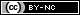# Gravity Gate

02 Окт. 2012
Posted by trektoArithmetic precision:* 512 bits Number of cycles: 55 000 Seconds per cycle: 0.005 Mass of (each) static objects: 1e13 kg. Mass of the moving object: 5e12 kg. Radius of each object: 20 m. Initial speed: 2 m/s Interacting law: Newton's law of gravity

*GMP is used for floating point numbers. Precision is compromised by math.h functions atan2, sin and cos. If you have C++ implementation of CORDIC or other fast algorithm, you can share it here.

More simulations

## Публикувай нов коментар

• Адресите на уеб-страници и e-mail адресите автоматично се конвертират в хипервръзки.
• Разрешени HTML tag-ове: <a> <p> <span> <div> <h1> <h2> <h3> <h4> <h5> <h6> <img> <map> <area> <hr> <br> <br /> <ul> <ol> <li> <dl> <dt> <dd> <table> <tr> <td> <em> <b> <u> <i> <strong><font> <del> <ins> <sub> <sup> <quote> <blockquote> <pre> <address> <code> <cite> <embed> <object> <param> <strike> <caption>
• Линиите и параграфите се прекъсват автоматично.
• Mark language-dependent sections with == lc == where lc (or lc-xx) is a language code, other or all.

Повече информация за опциите на форматиране